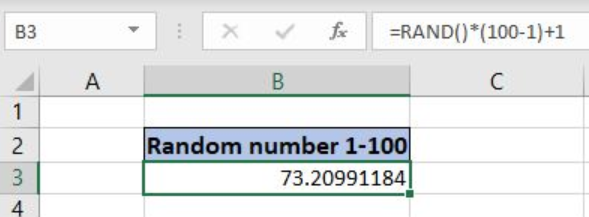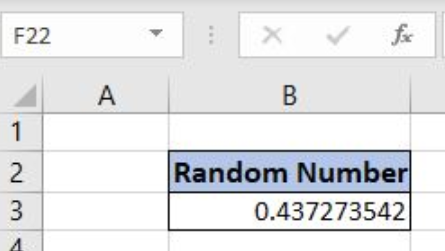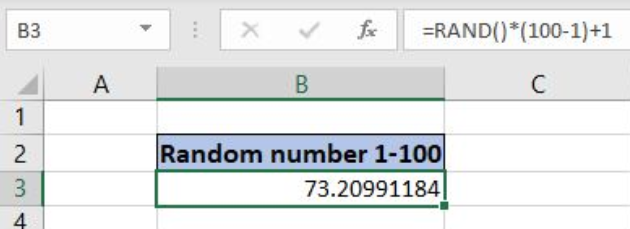Get instant live expert help with Excel or Google Sheets“My Excelchat expert helped me in less than 20 minutes, saving me what would have been 5 hours of work!”

#### Post your problem and you’ll get expert help in seconds.

Your message must be at least 40 characters
Our professional experts are available now. Your privacy is guaranteed.

# A Guide on Using the Excel Random Number Function

Excel allows a user to get a random decimal number between 0 and 1 by using the RAND function. We will also see how to make the function to return a number in any range. This step by step tutorial will assist all levels of Excel users in getting a random number.Figure 1. The final result of the RAND Function

## Syntax of the RAND Function

The generic formula is:

`=RAND()`

The function has no parameters.

## Get a Random Decimal Number between 0 and 1

We want to get a random decimal number between 0 and 1

The formula looks like:

`=RAND()`

To apply the RAND function, we need to follow these steps:

• Select cell B3 and click on it
• Insert the formula: `=RAND()`
• Press enterFigure 2. Get a random decimal number between 0 and 1

Every time we press enter in formula cell, we will get a new decimal number in B3.

## Get a Random Number between Two Numbers

We can modify the RAND function to make it return a random number between any two numbers. In our example, we want to get a random number between 1 and 100.

The formula looks like:

`=RAND() * (100 - 1) + 1`

We could put any two numbers in the formula, the generic formula looks like:

`=RAND() * (max - min) + min`

To apply the formula, we need to follow these steps:

• Select cell B3 and click on it
• Insert the formula: `=RAND() * (100 - 1) + 1`
• Press enterFigure 3. Get a random number between 1 and 100

Every time we press enter in formula cell, we will get a new decimal number between 1 and 100 in B3.

Most of the time, the problem you will need to solve will be more complex than a simple application of a formula or function. If you want to save hours of research and frustration, try our live Excelchat service! Our Excel Experts are available 24/7 to answer any Excel question you may have. We guarantee a connection within 30 seconds and a customized solution within 20 minutes.

Solution examplesI'm Apply a =RANDBETWEEN formula in one cells want to apply Formula to entire column and run formula when i hit the entire on the particular cell
Solved by M. S. in 20 minsHelp! In a coin toss I have a 1 in 2 (fifty-fifty) chance of getting heads or tails. I need a formula to determine my chance of getting 4 heads in a row or 4 tails in a row out of 10 coin tosses. If I get 4 in a row I win. What formula can I use to determine my chances of winning if I repeat the 10 tosses for hundreds or thousands of times? I get as far as =RANDBETWEEN(0,1) but I have not been able to figure out what formula to use to determine if there are 4 in a row in a group of 10 tosses, times thousands of groups with 10 tosses in each group. Thank-you for any help you can provide.
Solved by V. F. in 51 minsI have 4 column. when i fill the first 3 column. in 4th column i need a unique id number and i generate unique id number using RANDBETWEEN formula [ =CHAR(RANDBETWEEN(65,90))&CHAR(RANDBETWEEN(65,90))&RANDBETWEEN(1000,9900) ] but i want ..... when i'm filling the 3 column after that 4th column run this formula and generate unique id automatically
Solved by X. Q. in 20 minswhen i fill column A and B then C column generate Unique ID using RandBetween Formula {=CHAR(RANDBETWEEN(65,90))&CHAR(RANDBETWEEN(65,90))&RANDBETWEEN(1000,9900)} ...
Solved by S. Q. in 20 mins